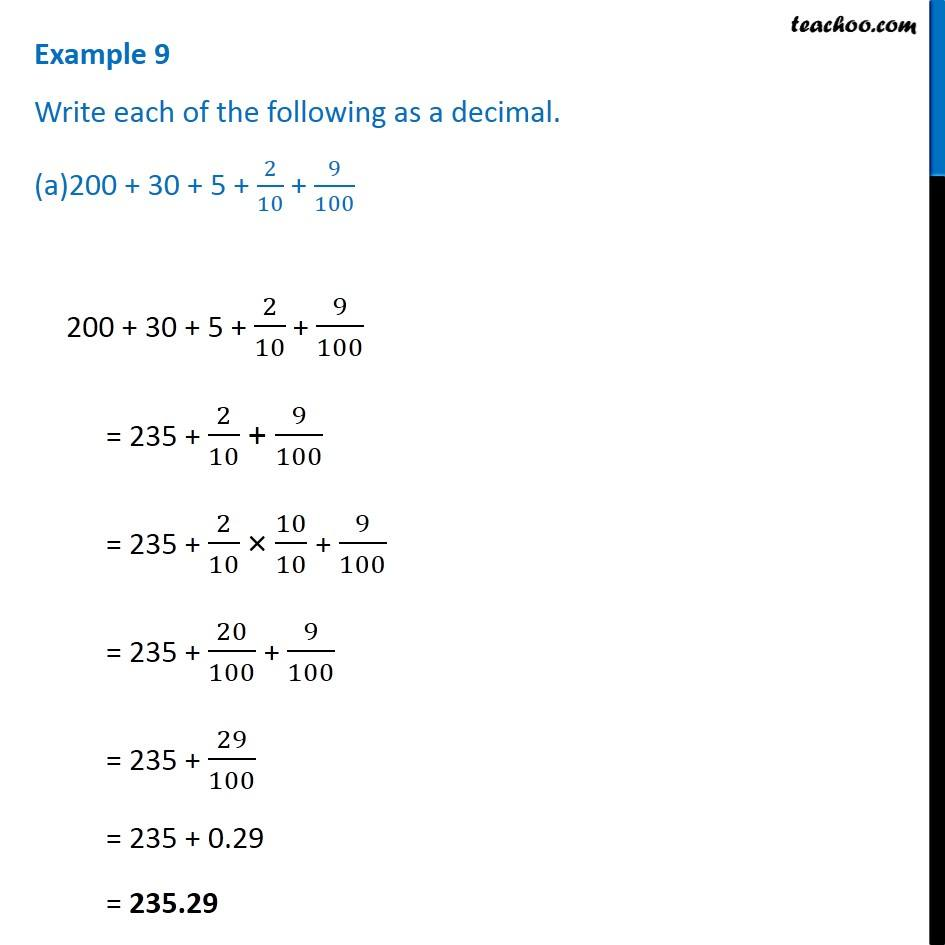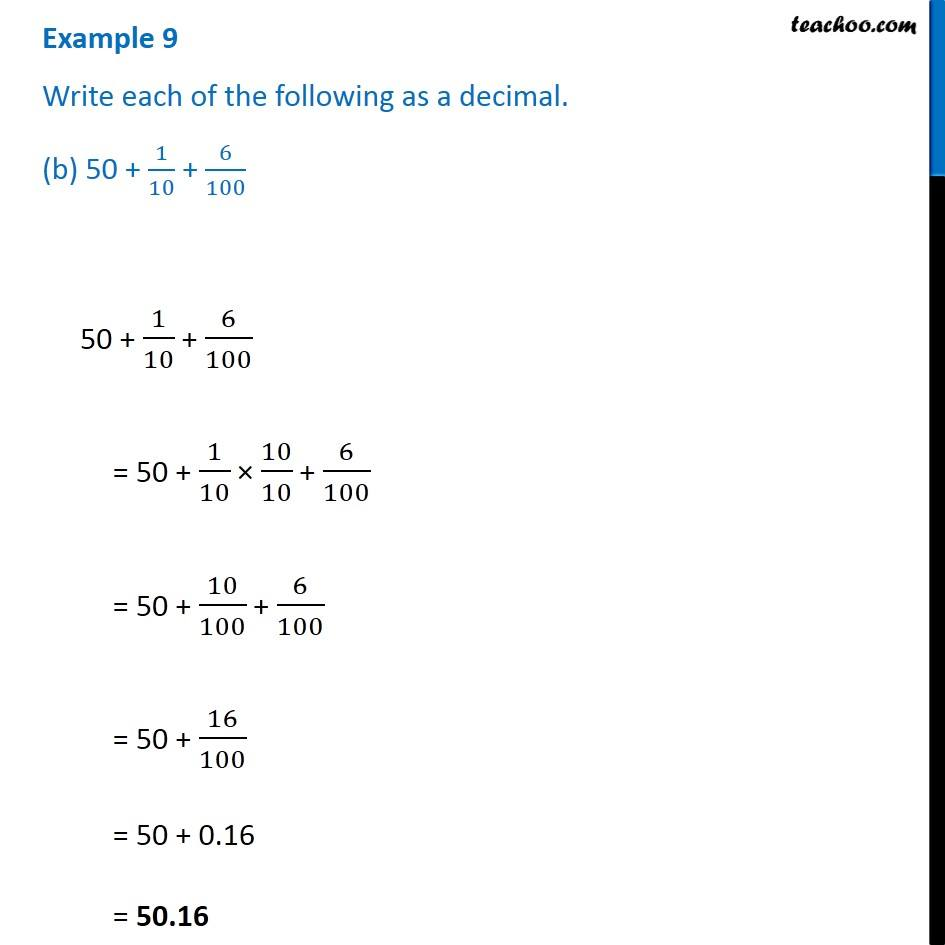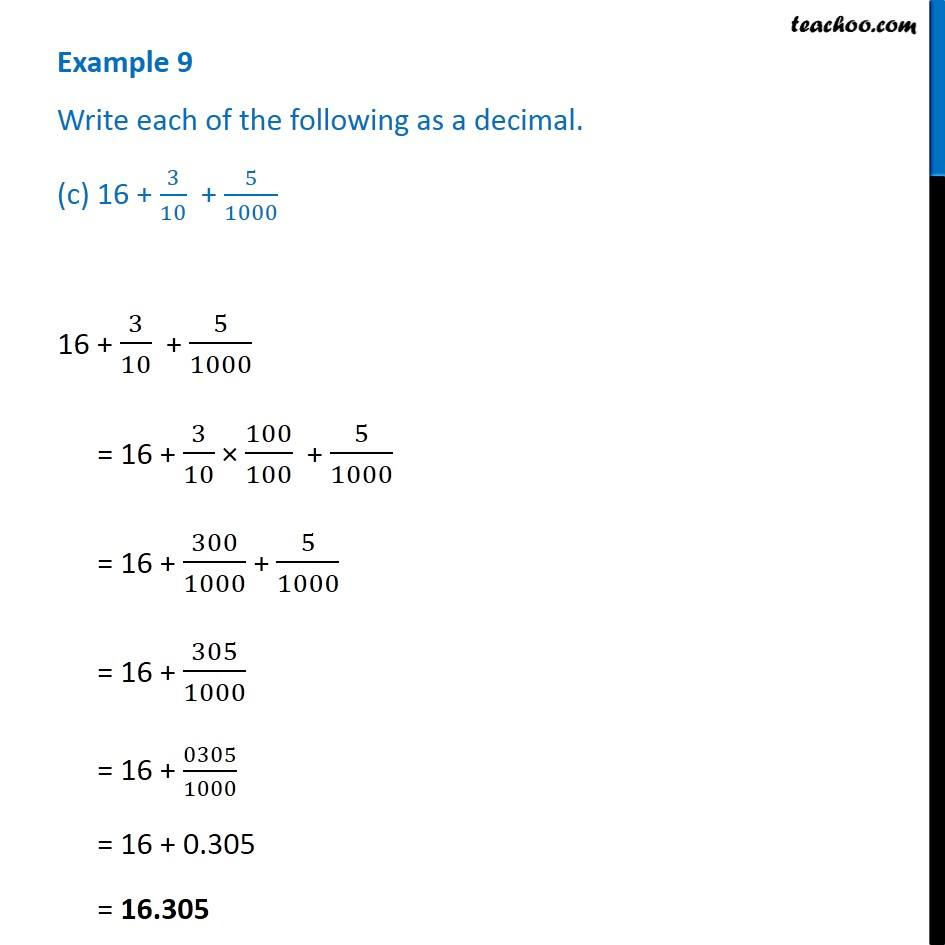1. Chapter 8 Class 6 Decimals
2. Serial order wise
3. Examples

Transcript

Example 9 Write each of the following as a decimal. (c) 16 + 3/10 + 5/1000 16 + 3/10 + 5/1000 = 16 + 3/10 × 100/100 + 5/1000 = 16 + 300/1000 + 5/1000 = 16 + 305/1000 = 16 + 0305/1000 = 16 + 0.305 = 16.305

Examples

Chapter 8 Class 6 Decimals
Serial order wise